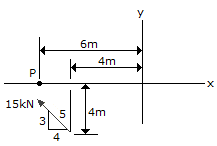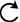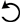# Engineering Mechanics - Force System Resultants - Discussion

### Discussion :: Force System Resultants - General Questions (Q.No.4)

4.Replace the force at A by an equivalent force and couple moment at P.

 [A]. F = 15 kN @= 36.9°, Mp = 67.1 kN-m[B]. F = 15 kN @= 36.9°, Mp = 30 kN-m[C]. F = 15 kN @= 143.1°, Mp = 67.1 kN-m[D]. F = 15 kN @= 143.1°, Mp = 30 kN-mExplanation:

No answer description available for this question.

 Channy said: (Mar 10, 2017) how that moment is calculated? Explain it.

 Tejas said: (Aug 5, 2017) Why option (A)? Explain.

 Babai Mukherjee said: (Jan 11, 2019) @All. 1st of all resolved the 15kN force. It should be 15 cos θ and 15 sin θ. And now look at the triangle given there you can determine the value of there. It should be Sin θ =3/4 and cos θ =5/4. Now, calculate the moment about pond p .so force is 15sin(3/4)*6 (6 is distant) = 67.5.

 Birhanu Biadge said: (Apr 6, 2021) Anyone explain this in detail.